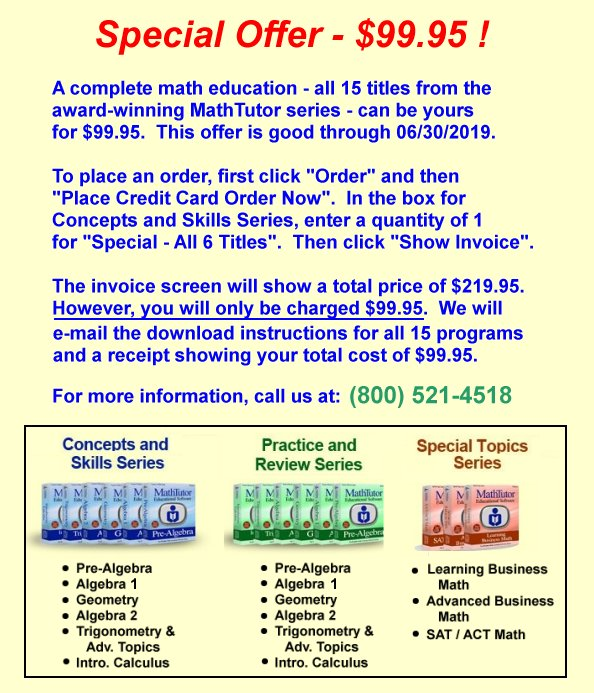Tutorial Screen - from "Order of Operations"

The screen shot seen below is from a lesson in our algebra 1 interactive tutorial program. Each lesson in the programs contains the following four sections:
Concept - This is a tutorial in which the concepts of the lesson are presented. Graphics and animation are utilized to enhance the presentation.
Examples - This section contains a variety of completely-worked examples to show how the concepts are applied. These examples prepare students to solve problems on their own.
Problems - An abundance of practice problems are contained in this section, enabling students to apply the concepts and skills they have learned. Students can increase the difficulty level of problems when they feel prepared to do so.
Test - This is a 5-problem test covering the material learned in the lesson. When a student answers 3 or more correctly (scores 60% or higher), they have successfully completed the learning objectives of the lesson.
The Navigation Panel, at the right edge of the screen, allows students to move directly to any of the four sections of a lesson. This is useful, for example, when a student has already worked in the lesson area and wishes to skip the concept and examples and go directly to practice problems or a lesson test.×